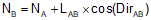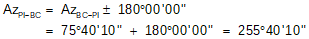### 4. Curves and Coordinates

#### a. Coordinate Equations

Equations C-16 and C-17 are general equations for computing coordinates using direction and distance from a known point, Figure C-26.Equation C-16Equation C-17Figure C-26. Coordinate Computation

Direction (Dir) may be either a bearing or azimuth.

Curve point coordinates can be computed using these equations from a base point. Since the radial chord method uses the BC as one end of all the chords, it can also be used as the base point for coordinate computations.

#### b. Computation Process

Assuming we start with the tangents and PI, then fit a curve, the general process is as follows:Figure C-27

The original tangent lines have directions; PC has coordinates.Figure C-28

A curve is fit to the tangents.

End points are at distance T from the PI along the tangents.Figure C-29

Compute coordinates of BC using back-direction of the tangent BC-PI and T.Equation C-18Equation C-19Figure C-30

Compute coordinates of EC using direction of the tangent PI-EC and T.

These will be use for a later math check.Equation C-20Equation C-21Figure C-31

Use a curve point's deflection angle to compute the direction if its radial chord from the BC.Equation C-22

δ is positive for right deflections, negative for left.
Using the direction and chord length, compute the point's coordinates.Equation C-23Equation C-24

#### c. Example

Continuing with the previous example problem.

Summary of given and computed curve data:

 Δ = 55°00'00" R = 500.00 ft D = 11°27'33.0" L = 479.965 ft T = 260.284 ft LC = 461.749 ft E = 63.691 ft M = 56.494 ft

 Point Station PI 25+00.00 BC 22+39.716 EC 27+19.681 Bk = 27+60.284 AhAdditional information: Azimuth of the initial tangent is 75°40'10"; coordinates of the PI are 1000.00 N, 5000.00' E.

Compute coordinates of the BC:Compute the coordinates of the EC:Set up Equatons C-21 through C-24 for this curve.This is the Radial Chord table computed previously:

 Curve Point Arc dist, li, (ft) Defl angle,δi Radial chord, c EC 27+19.681 Bk 479.965 27°30'00.0" 461.748 27+00 460.284 26°22'20.4" 444.203 26+00 360.284 20°38'33.9" 352.540 25+00 260.284 14°54'47.4" 257.355 24+00 160.284 9°11'01.0" 159.599 23+00 60.284 3°27'14.5" 60.248 BC 22+39.716 0.000 0°00'00.0" 0.000

Add three more columns for direction and coordinates:

 Curve Point Azimuth, Azi North, Ni East, Ei EC 27+19.681 Bk 27+00 26+00 25+00 24+00 23+00 BC 22+39.716

Complete the table using the three equations for this curve

At 22+39.716, we're still at the BC so the coordinates don't change.

At 23+00:At 24+00:and so on for the rest of the curve points.

The completed curve table is:

 Curve Point Azimuth, Azi North, Ni East, Ei EC 27+19.681 Bk 103°10'10.0 830.375 5197.419 27+00 102°02'30.4" 842.904 5182.244 26+00 96°18'43.9" 896.816 5098.218 25+00 90°34'57.4" 932.959 5005.157 24+00 84°51'11.0" 949.894 4906.770 23+00 79°07'24.5" 946.944 4806.981 BC 22+39.716 75°40'10" 935.576 4747.815

Math check: the coordinates computed for the EC in the table should be the same as the EC coordinates computed from the PI. Within rounding error, that's the case here.

#### d. Summary

The radial chord method lends itself nicely to computing curve point coordinates. The computations are not complex, although they are admittedly tedious.

Once coordinates are computed, field stakeout is much more flexible using Coordinate Geometry (COGO).